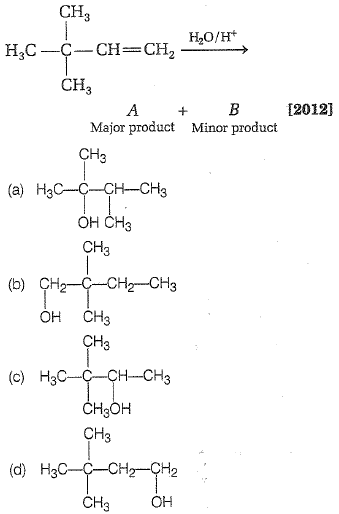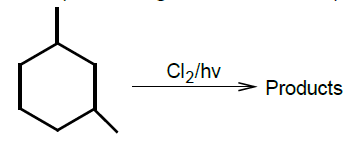Which is the most suitable reagent among the following to distinguish compound (III) from rest of the compounds?

I. CH3-C$\equiv$C-CH3

II. CH3-CH2-CH2-CH3

III. CH3-CH2-C$\equiv$CH

IV. CH3-CH=CH2

(a) Br2/CCl4                     (b) Br2/CH3COOH

(c)Alk.KMnO4                   (d) Ammonical AgNO3

Complete Question Bank + Test Series
Complete Question Bank

Difficulty Level:

Which of the following reagents will be able to distinguish between 1-butyne and 2-butyne?

(a) NaNH2            (b) HCl             (c) O2                 (d) Br2

Complete Question Bank + Test Series
Complete Question Bank

Difficulty Level:

A compound is treated with NaNH2 to give sodium salt. Identify the compound.

(a) C2H2                  (b) C6H6

(c) C2H6                   (d) C2H4

Complete Question Bank + Test Series
Complete Question Bank

Difficulty Level:

When chlorine is passed through propene at 400$°$C, which of the following is formed?

(a) PVC                         (b) Allyl chloride

(c) Nickel chloride          (d) 1,2-dichloro ethane

Complete Question Bank + Test Series
Complete Question Bank

Difficulty Level:

When acetylene is passed through $\mathrm{dil}.{\mathrm{H}}_{2}{\mathrm{SO}}_{4}$ in presence of ${\mathrm{HgSO}}_{4}$, the compound formed is

(a) ether                           (b) ketone

(c) acetic acid                    (d) acetaldehyde

Complete Question Bank + Test Series
Complete Question Bank

Difficulty Level:

Benzene reacts with ${\mathrm{CH}}_{3}\mathrm{Cl}$ in the presence of anhy. ${\mathrm{AlCl}}_{3}$ to form

(a) toluene

(b) chlorobenzene

(c) benzylchloride

(d) xylene

Complete Question Bank + Test Series
Complete Question Bank

Difficulty Level:

In the following reaction,Complete Question Bank + Test Series
Complete Question Bank

Difficulty Level:

2-bromopentane is heated with potassium ethoxide in ethanol. The major product obtained is

(a) 2-ethoxypentane                    (b)pentene-1

(c) trans-pentene-2                     (d) cis -pentene-2

Complete Question Bank + Test Series
Complete Question Bank

Difficulty Level:

CH3-C$\equiv$C-H$\stackrel{{\mathrm{CH}}_{3}\mathrm{MgBr}}{\to }$CH4 + (A)(B); (B) will be

(1) H3C-CH2-CH2-COOH                                (2) CH3-C$\equiv$C-CH3

(3) H3C-C$\equiv$C-COOH                                      (4) H3C-CH=CH-COOH

Complete Question Bank + Test Series
Complete Question Bank

Difficulty Level:

The monochlorinated products (excluding stereo-isomers) obtained from the reaction is:(1) 4            (2) 5              (3) 6            (4) 7